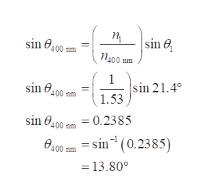# Using filters, a photographer has created a beam of light that consists of three wavelengths: 400 nm (violet), 500 nm (green), and 650 nm (red). He aims the beam so that it passes through air and then enters a block of crown glass. The beam enters the glass at an incidence angle ofθ1 = 21.4°.The glass block has the following indices of refraction for the respective wavelengths in the light beam.wavelength (nm)400500650index of refractionn400 nm = 1.53n500 nm = 1.52n650 nm = 1.51(a)Upon entering the glass, are all three wavelengths refracted equally, or is one bent more than the others? (b)What are the respective angles of refraction (in degrees) for the three wavelengths? (Enter each value to at least two decimal places.)(i)θ400 nm°(ii)θ500 nm°(iii)θ650 nm°

Question
34 views
Using filters, a photographer has created a beam of light that consists of three wavelengths: 400 nm (violet), 500 nm (green), and 650 nm (red). He aims the beam so that it passes through air and then enters a block of crown glass. The beam enters the glass at an incidence angle of
θ1 = 21.4°.
The glass block has the following indices of refraction for the respective wavelengths in the light beam.
 wavelength (nm) index of refraction 400 500 650 n400 nm = 1.53 n500 nm = 1.52 n650 nm = 1.51

(a)
Upon entering the glass, are all three wavelengths refracted equally, or is one bent more than the others?

(b)
What are the respective angles of refraction (in degrees) for the three wavelengths? (Enter each value to at least two decimal places.)

(i)
θ400 nm
°
(ii)
θ500 nm
°
(iii)
θ650 nm
°

check_circle

Step 1

(a)

From Snell’s law,

Step 2

From above relation, it is clear that, for the same incident angle, the refracted angle will be different. Higher will be the index of refraction (n2), lower will be the refracted angle.

Therefore, all the wavelengths will not bent same instead one will bent more than others.

Step 3

(b)

(i)

For air index of refraction is 1.

Rewrite the expressio...help_outlineImage Transcriptionclosesin 8200 -m sin e N00 nm sin 21.4° 1.53 sin 6,00- sin O400 pm = 0.2385 e.00 m = sin* (0.2385) = 13.80° fullscreen

### Want to see the full answer?

See Solution

#### Want to see this answer and more?

Solutions are written by subject experts who are available 24/7. Questions are typically answered within 1 hour.*

See Solution
*Response times may vary by subject and question.
Tagged in

### Ray Optics